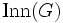# Abelian automorphism group implies class two

This article gives the statement and possibly, proof, of an implication relation between two group properties. That is, it states that every group satisfying the first group property (i.e., group whose automorphism group is abelian) must also satisfy the second group property (i.e., group of nilpotency class two)
View all group property implications | View all group property non-implications
Get more facts about group whose automorphism group is abelian|Get more facts about group of nilpotency class two
This article gives a result about how information about the structure of the automorphism group of a group (abstractly, or in action) can control the structure of the group
View other such results

## Statement

Let$G$ be a group whose automorphism group is abelian, i.e., the automorphism group of$G$ is an abelian group. Then,$G$ is a group of nilpotency class two

## Proof

Since$\operatorname{Aut}(G)$ is an abelian group, the subgroup$\operatorname{Inn}(G)$, the inner automorphism group is also an abelian group. But$\operatorname{Inn}(G) \cong G/Z(G)$, where$Z(G)$ is the center of$G$. Thus,$G/Z(G)$ is abelian, so$G$ has class two.